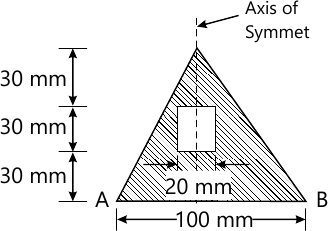MORE IN Elements of Civil Engg. & Engg. Mechanics
VTU First Year Engineering (P Cycle) (Semester 2)
Elements of Civil Engg. & Engg. Mechanics
December 2012
Total marks: --
Total time: --
INSTRUCTIONS
(1) Assume appropriate data and state your reasons
(2) Marks are given to the right of every question
(3) Draw neat diagrams wherever necessary

Choose the correct answer for the following :-
1 (a) (i) A branch of civil engineering that deals with testing soils and foundation design is called
(A) Geotechnical engineering
(B) Structural engineering
(C) Environmental engineering
(D) Highway engineering
1 M
1 (a) (ii) Highways which are superior to National Highways and are provided whatever volume of traffic is very high are :
(A) Airways
(B) Expressways
1 M
1 (a) (iii) Composite material made using cement concrete and steel is called:
(A) Plain cement concrete
(B) Composite cement concrete
(C) Reinforced cement concrete
(D) Prestressed cement concrete
1 M
1 (a) (iv) A bridge constructed at some angle to river flow is
(A) Bascule bridge
(B) Square bridge
(C) RCC bridge
(D) Skew bridge
1 M
1 (b) Write a note on impact of infrastructural development on the economy of the country.
8 M
1 (c) Explain different types of dams, with neat sketches.
8 M

Choose the correct answer for the following :-
2 (a) (i) Forces whose line of action lie along the same line are:
(A) Coplanar non-concurrent
(B) Coplanar parallel
(C) Colinear
(D) Concurrent
1 M
2 (a) (ii) Moment of a force about a moment centre is the measure of its
(A) Rotational effect
(B) Translatory effect
(C) Both A and B
(D) None of these
1 M
2 (a) (iii) The translatory effect of a couple on the rigid body is,
(A) Zero
(B) Maximum
(C) Minimum
(D) None of these
1 M
2 (a) (iv) An object with only mass but no size in mechanics is,
(A) Rigid body
(B) Point body
(C) Particle
(D) Deformable body
1 M
2 (b) State and prove Varignon's theorem.
8 M
2 (c) (i) A force of 200 N is acting on a block shown in Fig. Q2(c) (i). Find the components of forces along horizontal and vertical axes. Ignore the friction between contact surfaces.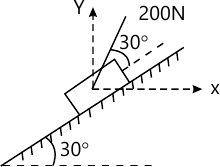4 M
2 (c) (ii) A nail is to be removed by applying 4 kN force and a force 'F' as shown in Fig.Q2(c) (ii). Find the magnitude of forces 'F' so that nail is pulled out vertically from the ground. Also, determine the resulting pull along vertical axis.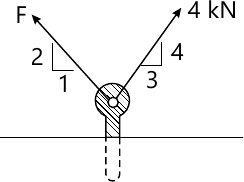4 M

Choose the correct answer for the following :-
3 (a) (i) If two concurrent forces each of P act at right angles to each other, their resultant will be equal to,
(A) P?2
(B) 2?P
(C) 4P
(D) P
1 M
3 (a) (ii) The technical of finding the resultant of a system of forces is called,
(A) Composition
(B) Resolution
(C) Equilibrium
(D) None of these
1 M
3 (a) (iii) In a coplanar concurrent force system if ?V+0, then the resultant is,
(A) Moment of the force system
(B) Vertical
(C) Horizontal
(D) None of these
1 M
3 (a) (iv) if two forces act at an angle of 120°, the greater force is 50 N and their resultant is perpendicular to smaller force, the magnitude of smaller force is,
(A) 43.33 N
(B) 32.50 N
(C) 25 N
(D) None of these
1 M
3 (b) Determine the resultant force acting on the structure at point 'O' both in magnitude and direction for the system of forces shown in Fig.Q3 (b).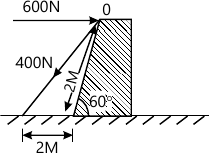6 M
3 (c) Determine the magnitude, direction and point of application of the resultant force for the system of forces show in Fig.Q3 (c) with respect to point 'O'.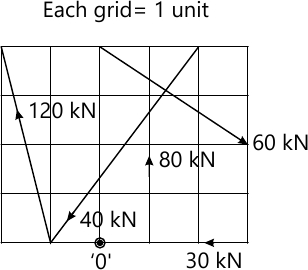10 M

Choose the correct answer for the following :-
4 (a) (i) Centroid of plane is the point at which,
(A) Weight of the body at which,
(B) Mass of the body is connected
(C) Surface area of the body is connected
(D) All of these
1 M
4 (a) (ii) An axis over which one half of the plane figure is just the mirror of the other half is called:
(A) Bottom axis
(B) Axis of symmetry
(C) Unsymmetrical axis
(D) All of these
1 M
4 (a) (iii) The centroid of the plane lamina will not be at its geometrical centre if it is a:
(A) Rectangle
(B) Square
(C) Circle
(D) Right angle triangle
1 M
4 (a) (iv) Centroid of a quarter of circular lamina lies from diameter line at a distance of:
(A) 4R/3?
(B) R/3?
(C) 2R/3?
(D) None of these
1 M
4 (b) Locate the centroid of a triangle by the method of integration.
6 M
4 (c) Locate the centroid of the shaded area shown in Fig. Q4(c) with respect OX and OY. All dimensions are in mm.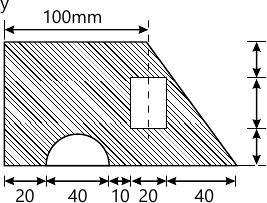10 M

Choose the correct answer for the folowing :-
5 (a) (i) A free body diagram is a diagram.
(A) Drawn by free hand
(B) Represents a floating body
(C) Separating the body from its surrounding and replacing with force vector
(D) All of these
1 M
5 (a) (ii) The Lami's theorem can be applied only when number of unknown forces are:
(A) Two
(B) Three
(C) Five
(D) None of these
1 M
5 (a) (iii) If a body is in equilibrium , it is concluded that,
(A) No force is acting
(B) Resultant is zero
(C) Moment about any point is zero
(D) Both A and B
1 M
5 (a) (iv) For a smooth sperical surface reaction acts:
(A) Horizontal to plane of contact
(B) Inclined to plane of contact
(C) Perpendicular to plane of contact
(D) None of these
1 M
5 (b) An electric bulb weighing 150N is suspended between wall and the roof by two wires as shown in Fig.Q5(b). Determine the tension in the wires using Lami's theorem.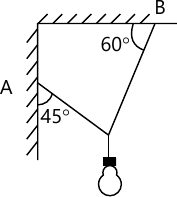6 M
5 (c) Find the reaction at the contact surface for two identical cylinders weighning 1000N each as shown in Fig.Q5(c).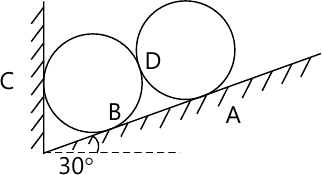10 M

Choose the correct answer for the following :-
6 (a) (i) Reaction line at roller support with respect to plane of contact is,
(A) Oblique
(B) Perpendicular
(C) Inclined
(D) None of these
1 M
6 (a) (ii) When a load acts at constant rate over given length of a beam is called,
(B) udl
(C) uvl
(D) All of these
1 M
6 (a) (iii) At the fixed end of Cantilever, the number of unknowns reaction components are:
(A) 1
(B) 2
(C) 3
(D) 4
1 M
6 (a) (iv) Minimum number of members required to from a simple truss is
(A) 2
(B) 3
(C) 4
(D) 5
1 M
6 (b) Define perfect and imperfect truss. Hence list the assumptions made in the analysis of simple truss.
6 M
6 (c) Determine the reaction components for the loaded beam shwon in Fig.Q6(c).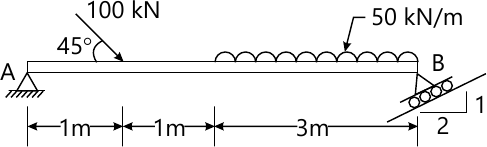10 M

Choose the correct answer for the following :-
7 (a) (i) The maximum frictional force developed when the body just begins to slide is called:
(A) Limiting friction
(B) Rolling friction
(C) static friction
(D) None of these
1 M
7 (a) (ii) Compared to static friction, kinetic friction is,
(A) Larger
(B) Equal
(C) Smaller
(D) None of these
1 M
7 (a) (iii) Angle of friction is the angle between,
(A) Normal reaction and friction force
(B) Normal reaction and resultant
(C) Weight of the body and friction force
(D) Normal reaction and weight of the body
1 M
7 (a) (iv) The force of friction depends on:
(A) Area of contact
(B) Roughness of contact surface
(C) Both A and B
(D) None of these
1 M
7 (b) Explain briefly :-
(i) Angle of repose
(ii) Cone of friction
6 M
7 (c) A ladder weighing 200 N is supported as shown in Fig.Q7(c), if a man weighing 650 N climbs to the top of the ladder, determine the inclination of the ladder with the floor at which the ladder is to be placed to prevent slipping. Take ?=0.25 for all contact surfaces.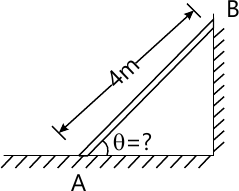10 M

Choose the correct answer for the following :-
8 (a) (i) Moment of inertia is,
(A) Second moment of area
(B) First moment of area
(C) Third moment of area
(D) None of these
1 M
8 (a) (ii) M.I. of circular section about centroidal axis is,
(A) ?D4/32
(B) ?D4/48
(C) ?D4/64
(D) ?D4/128
1 M
8 (a) (iii) The unit of radius of gyration is,
(A) mm
(B) mm2
(C) mm3
(D) mm4
1 M
8 (a) (iv) M.I. of a square of side 'B' about its centroidal axis is,
(A) B4/8
(B) B4/12
(C) B4/36
(D) B4/48
1 M
8 (b) State and prove parallel axis theorem :
6 M